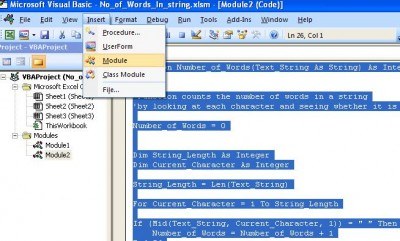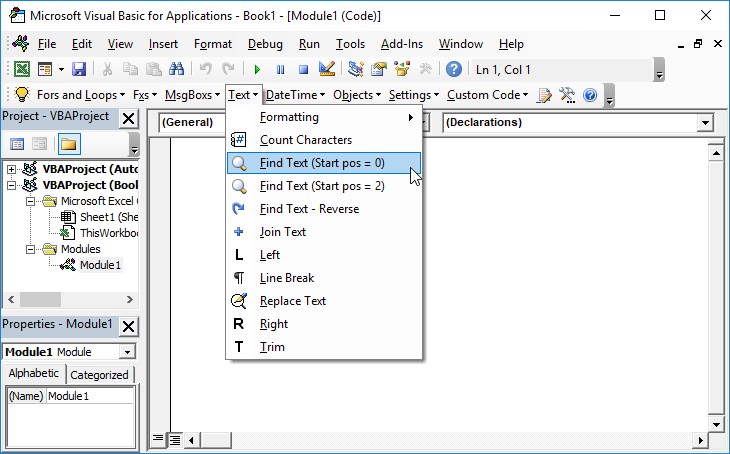# VBA Function To Calculate Number of Words in a String

## Count Number of Words in a String

The following VBA function counts the number of words in a string by searching for spaces within the string. It uses the MID Function. You could accomplish something similar with the SEARCH or FIND Functions.

``````Function Number_of_Words(Text_String As String) As Integer
'Function counts the number of words in a string
'by looking at each character and seeing whether it is a space or not
Number_of_Words = 0
Dim String_Length As Integer
Dim Current_Character As Integer

String_Length = Len(Text_String)

For Current_Character = 1 To String_Length

If (Mid(Text_String, Current_Character, 1)) = " " Then
Number_of_Words = Number_of_Words + 1
End If

Next Current_Character
End Function``````

It is worth noting a couple of points:
• This code must be inserted in a Workbook Module – from the VBA pane, click on Insert and then module• The function can be called from an excel workbook cell either with an either explicit text or cell reference as an argument:
A3 = Number_of_Words(“Pig Dog Cat”)
A4 = Number_of_Words(D1)

The function works by traversing the length of a string and seeing if the next character is a space and if so it adds one to the number of spaces in the string.